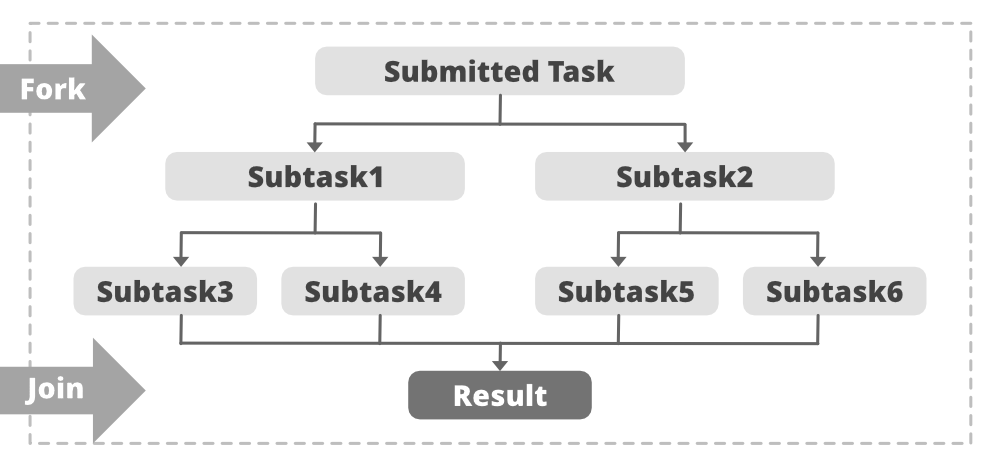Open in App
Not now

# ForkJoinPool Class in Java with Examples

• Difficulty Level : Hard
• Last Updated : 07 Jun, 2021

The ForkJoinPool class is the center of the fork/join framework, which is an implementation of the ExecutorService interface. ForkJoinPool class is an extension of the AbstractExecutorService class, and it implements the work-stealing algorithm (i.e., worker threads that run out of things to do can steal tasks from other threads that are still busy) of fork/join framework and can execute ForkJoinTask processes.

The ForkJoinPool class inherits the following methods from java.util.concurrent.AbstractExecutorService class:

• invokeAll()
• invokeAny()

The ForkJoinPool class inherits the following methods from Methods inherited from class java.lang.Object class:

• clone()
• equals()
• finalize()
• getClass()
• hashCode()
• notify()
• notifyAll()
• wait()

Syntax:

`public class ForkJoinPool extends AbstractExecutorService  `

Join: After the execution of the subtasks, the task may join all the results into one result.

This is illustrated in the diagram below:Example:

getActiveThreadCount(): This method returns an estimated number of threads that are currently stealing or executing tasks. It may overestimate the number of active threads.

Syntax

`public int getActiveThreadCount()`

## Java

 `// Java program to demonstrate the``// Implementation of getActiveThreadCount()` `import` `java.util.ArrayList;``import` `java.util.List;``import` `java.util.concurrent.ForkJoinPool;``import` `java.util.concurrent.RecursiveAction;``class` `NewTask ``extends` `RecursiveAction``{``    ``private` `long` `Load = ``0``;``    ` `    ``public` `NewTask(``long` `Load) { ``this``.Load = Load; }` `    ``protected` `void` `compute()``    ``{``        ``// fork tasks into smaller subtasks``        ``List subtasks = ``new` `ArrayList();``        ``subtasks.addAll(createSubtasks());``        ` `        ``for` `(RecursiveAction subtask : subtasks) {``            ``subtask.fork();``        ``}``    ``}``    ` `    ``// function to create and add subtasks``    ``private` `List createSubtasks()``    ``{``        ``// create subtasks``        ``List subtasks = ``new` `ArrayList();``        ``NewTask subtask1 = ``new` `NewTask(``this``.Load / ``2``);``        ``NewTask subtask2 = ``new` `NewTask(``this``.Load / ``2``);``        ``NewTask subtask3 = ``new` `NewTask(``this``.Load / ``2``);``        ` `        ``// to add the subtasks``        ``subtasks.add(subtask1);``        ``subtasks.add(subtask2);``        ``subtasks.add(subtask3);``        ` `        ``return` `subtasks;``    ``}``}``public` `class` `JavaForkJoingetActivethreadcountExample1 {``    ``public` `static` `void` `main(``final` `String[] arguments)``        ``throws` `InterruptedException``    ``{``        ``// get no. of available core available``        ``int` `proc = Runtime.getRuntime().availableProcessors();``        ` `        ``System.out.println(``"Number of available core in the processor is: "``            ``+ proc);``            ` `        ``// get no. of threads active``        ``ForkJoinPool Pool = ForkJoinPool.commonPool();``        ` `        ``System.out.println(``"Number of active thread before invoking: "``            ``+ Pool.getActiveThreadCount());``            ` `        ``NewTask t = ``new` `NewTask(``400``);``        ` `        ``Pool.invoke(t);``        ` `        ``System.out.println(``"Number of active thread after invoking: "``            ``+ Pool.getActiveThreadCount());``        ``System.out.println(``"Common Pool Size is: "``                           ``+ Pool.getPoolSize());``    ``}``}`

Output

```Number of available core in the processor is: 4
Number of active thread before invoking: 0
Number of active thread after invoking: 3
Common Pool Size is: 3```

### Methods of ForkJoinPool Class

My Personal Notes arrow_drop_up